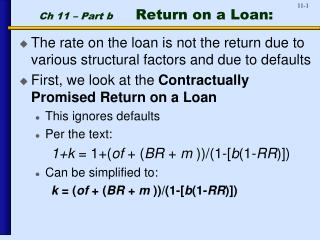DownloadDownload PresentationCh 11 – Part b Return on a Loan:

# Ch 11 – Part b Return on a Loan:

Download Presentation## Ch 11 – Part b Return on a Loan:

- - - - - - - - - - - - - - - - - - - - - - - - - - - E N D - - - - - - - - - - - - - - - - - - - - - - - - - - -
##### Presentation Transcript

1. Ch 11 – Part b Return on a Loan: • The rate on the loan is not the return due to various structural factors and due to defaults • First, we look at the Contractually Promised Return on a Loan • This ignores defaults • Per the text: 1+k = 1+(of + (BR + m ))/(1-[b(1-RR)]) • Can be simplified to: k = (of + (BR + m ))/(1-[b(1-RR)])

2. Contractually Promised Return on a Loan: • Factors that determine the stated rate on the loan: • BR= base rate. Could be the prime rate or Libor • m = Margin. The margin in excess of the base rate. • BR + m = stated rate

3. Contractually Promised Return on a Loan: • Factors that add to the profitability of the loan: • of = Origination fees. Note that there are some simplifying assumptions in the way that these are treated. First, the we just add them in, we are making the implicit assumption either that the loan is for one or that we collect the fees each year. Second we actually receive them at origination but they are treated the same as year-end cash flows. • b = compensating balance. This is a non-interest bearing deposit the borrower must maintain at the bank for the duration of the loan. This improves profitability by essentially requiring less funds to lend while collecting interest on the gross amount.

4. Contractually Promised Return on a Loan: • Factor that reduces profitability of the loan: • RR = Reserve requirement. This factor has a very minor impact on the return. Recall that reserves are required to be held against deposits, not loans. The reserve requirement that is included in this calculation is the reserve amount required for the deposit received as a compensating balance (if there is one). http://www.bloomberg.com/apps/cbuilder?ticker1=ARDIERNA%3AIND

5. Contractually Promised Return on a Loan: • Back to the calculation: • k = (of + (BR + m ))/(1-[b(1-RR)]) • k = (income received) / (money lent)

6. Sensitivity to Money Lent Factors

7. Loan Er: Now Include Defaults • Er = (1 + k)*P + (0)*(1-P)*(1+k) -1 Where P= probability of complete repayment of loan, so (1-P) is probability of default • Er = (1 + k)*P + (0)*(1-P)*(1+k) - 1 Collected if loan fully paid • Er = (1 + k)*P + (0)*(1-P)*(1+k) -1 Recovery in default • Note that text never varies from the assumption that recoveries = 0%. We will investigate the impact of recoveries.